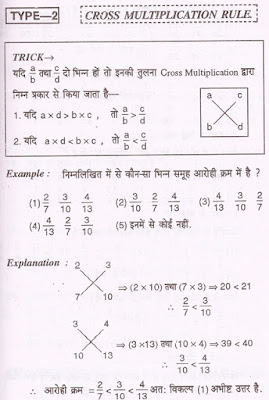भिन्नो की तुलना: Maths Comparison of Fractions Short Tricks in Hindi भिन्नो की तुलना - Online Solution, Maths Short Tricks, Educational, Mobile Features, Toll Free, customer care number

# भिन्नो की तुलना: Maths Comparison of Fractions Short Tricks in Hindi भिन्नो की तुलना

## भिन्नो की तुलना Comparison of Fractions

In this article we share some important short tricks of fractions questions in Hindi as well as English. These tricks is very useful for those learners who want to crack various national and state level competitive exams. We also share some basic definition, types of questions and most important examples which is asked in previous year exams.

## If (a x d) > (b x c),      then   a/b > c/d If (a x d) < (b x c),      then   a/b < c/d Example :- Which of the following fraction is in ascending order ? A) 2/7     3/10     4/13    B) 3/10    2/7      4/13     C) 4/13    3/10    2/7  D) 4/13    2/7      3/10Please click for More >>> Maths Short Tricks in Hindi

## TRICK :-

### L.C.M rule

1. If in given fraction L.C.M. of numerator is smaller then the L.C.M. of there denominator then the numerator and denominator of fraction multiplied by suitable number to equalize numerator with the L.C.M. Now the fraction whose denominator is smallest will be the biggest fraction and the fraction whose denominator is biggest is consider as the smallest fraction.

1. If in given fraction L.C.M. of denominator is smaller then the L.C.M of numerator , then the numerator and denominator of fraction multiplied by suitable number to equalize denominator  with the L.C.M. Now the fraction whose denominator is smallest will be the smallest fraction and the fraction whose denominator is biggest is consider as the biggest fraction.

## TRICK :-

### Some fraction are easier to compare by division method. In such type of fractions numerator is divided by its denominator sequentially and resultant is got by comparing the quotient.

Example :- Which of the following fraction is consider as biggest ?
A) 9/17        B) 8/15        C) 5/12            D) 7/13         E) 4/9